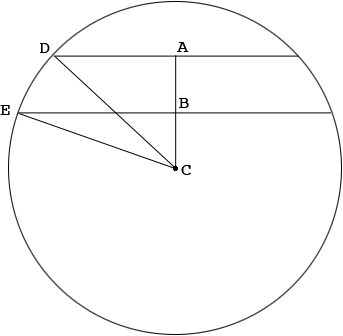SEARCH HOMEMath Central Quandaries & QueriesQuestion from Ella: In a circle, a 30 unit chord is 5 units closer to the center than a 20 unit chord. Find the radius.Hi Ella,

In my diagram below$A$ and $B$ are the midpoints of the two chords and $C$ is the center of the circle. The distance from $A$ to $B$ is 5 units and $CD$ and $CE$ are radii of the circle. Suppose the length of $BC$ is $x$ units and the radius is $r$ units.Triangles $ADC$ and $BEC$ are right triangles and hence the use of Pythagoras theorem on them gives two equations in the variables $x$ and $r.$ Solve for $x$ and then $r.$

Penny* Registered trade mark of Imperial Oil Limited. Used under license.Math Central is supported by the University of Regina and the Imperial Oil Foundation.